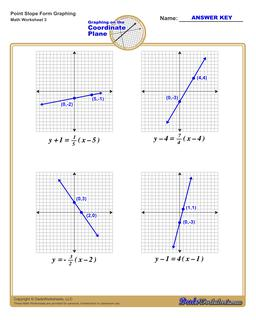PLEASE GO BACK AND USE THE BIG BLUE 'PRINT' BUTTON ON THE PAGE TO PRINT THE WORKSHEET CORRECTLY!Sorry for the trouble! The browser won't print the embedded worksheet PDF directly using the normal 'Print' command in the file menu, so you need to click the big 'Print' button to send just the worksheet and not the surrounding page to the printer.

# Math Worksheets: Linear Equations: Linear Equations: Graph Point Slope Form (Third Worksheet)## Graph Point Slope Form (Third Worksheet)

Property Value
DescriptionGraph Point Slope Form: Each graphing linear equations worksheet on this page has four coordinate planes and equations in point-slope form, and includes an answer key showing the correct graph. (Third Worksheet)
Resource TypeWorksheet